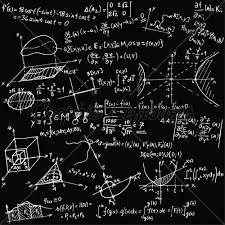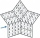# Unknown number

If I reduced the sum of the numbers 70 and the unknown number three times, I would get 100. what is the unknown number?

x =  230

### Step-by-step explanation:

(70+x)/3 = 100

x = 230

Our simple equation calculator calculates it.Did you find an error or inaccuracy? Feel free to write us. Thank you!Tips to related online calculators
Need help to calculate sum, simplify or multiply fractions? Try our fraction calculator.
Do you have a linear equation or system of equations and looking for its solution? Or do you have a quadratic equation?
Do you want to perform natural numbers division - find the quotient and remainder?

## Related math problems and questions:

• Unknown number 5I think of an unknown number. If we enlarge it five times, then subtract 3, and the result decreases by 75%, we get one greater than the number. What number am I thinking of?
• Unknown number4/5 of a number is 276. what is 2/3 of the same number?
• Unknown number 716% of the unknown number is by 21 less than unknown number itself. Determine the natural unknown number.
• Unknown numberSamuel wrote unknown number. Then he had add 200000 to the number and the result multiply by three. When it calculated he was surprised, because the result would have received anyway, if write digit to the end of original number. Find unknown number.
• UN 1If we add to an unknown number his quarter, we get 210. Identify unknown number.
• Unknown xIf we add to unknown number 21, then divide by 6 and then subtract 51, we get back an unknown number. What is this unknown number?
• Expressions with variableThis is algebra. Let n represent an unknown number and write the following expressions: 1. 4 times the sum of 7 and the number x 2. 4 times 7 plus the number x 3. 7 less than the product of 4 and the number x 4. 7 times the quantity 4 more than the number
• Two numbersWe have two numbers. Their sum is 140. One-fifth of the first number is equal to half the second number. Determine those unknown numbers.
• Unknown numberFind the unknown number equal to a quarter of a fifth of a number, which is by 152 more than an unknown number.
• Guess numberWith what number should be divided, number 9727 to be reduced by 70%?
• One-thirdA one-third of unknown number is equal to five times as great as the difference of the same unknown number and number 28. Determine the unknown number.
• RemaindersIt is given a set of numbers { 170; 244; 299; 333; 351; 391; 423; 644 }. Divide this numbers by number 66 and determine set of remainders. As result write sum of this remainders.
• Prime divisorsFind 2/3 of the sum's ratio and the product of all prime divisors of the number 120.
• Unknown numberFind an unknown number of which 1/5 is 40 greater than one-tenth of that number.
• FractionsThree-quarters of an unknown number is 4/5. What is 5/6 of this unknown number?
• All pairsDetermine all pairs (m, n) of natural numbers for which is true: m s (n) = n s (m) = 70, where s (a) denotes the digit sum of the natural number a.
• The number 72The number 72 increase by 25%. By how much % will you have to reduce the number you created to get the number 72 again?